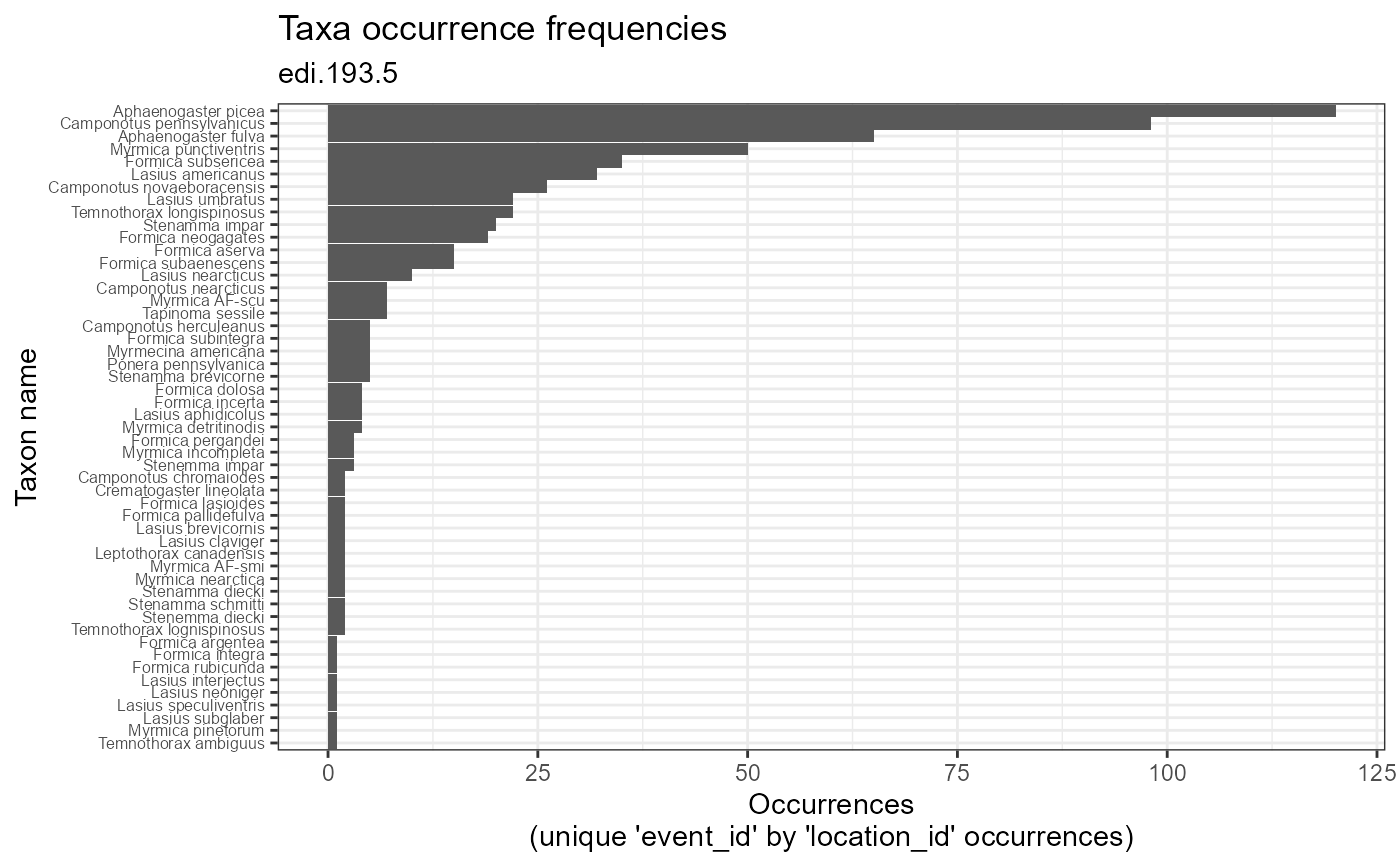Plot taxon occurrence frequences as the number of 'event_id' by 'location_id' combinations in which a taxon is observed.

plot_taxa_occur_freq(
data,
id = NA_character_,
min_occurrence = 0,
facet_var = NA_character_,
color_var = NA_character_,
facet_scales = "free",
alpha = 1
)

## Arguments

data

(list or tbl_df, tbl, data.frame) The dataset object returned by read_data(), a named list of tables containing the observation and taxon tables, or a flat table containing columns of the observation and taxon tables.

id

(character) Identifier of dataset to be used in plot subtitles. Is automatically assigned when data is a dataset object containing the id field, or is a table containing the package_id column.

min_occurrence

(numeric) Minimum number of occurrences allowed for taxa included in the plot.

facet_var

(character) Name of column to use for faceting. Must be a column of the observation or taxon table.

color_var

(character) Name of column to use for plot colors.

facet_scales

(character) Should scales be free ("free", default value), fixed ("fixed"), or free in one dimension ("free_x", "free_y")?

alpha

(numeric) Alpha-transparency scale of data points. Useful when many data points overlap. Allowed values are between 0 and 1, where 1 is 100% opaque. Default is 1.

## Value

(gg, ggplot) A gg, ggplot object if assigned to a variable, otherwise a plot to your active graphics device.

## Details

The data parameter accepts a range of input types but ultimately requires the 13 columns of the combined observation and taxon tables.

## Examples

if (FALSE) {
# Read a dataset of interest

# Plot the dataset
plot_taxa_occur_freq(dataset)

# Facet by location and color by taxon_rank
plot_taxa_occur_freq(
data = dataset,
facet_var = "location_id",
color_var = "taxon_rank")

# Color by location and only include taxa with >= 5 occurrences
plot_taxa_occur_freq(
data = dataset,
color_var = "location_id",
min_occurrence = 5)

# Flatten, filter using a time cutoff, then plot
dataset %>%
flatten_data() %>%
dplyr::filter(lubridate::as_date(datetime) > "2003-07-01") %>%
plot_taxa_occur_freq()
}
# Plot the example dataset
plot_taxa_occur_freq(ants_L1)